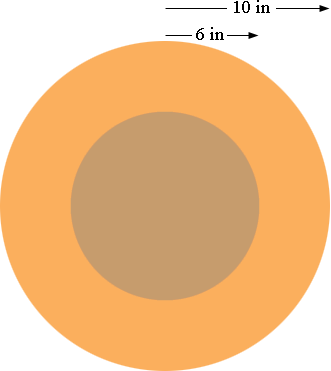SEARCH HOMEMath Central Quandaries & QueriesQuestion from maddy, a student: Find the exact area of the region bounded by two concentric circles with radii 10 inches and 6 inches.The area of a circle of radius r inches is π r2 square inches. Concentric circles have the same centre.What is the area of a circle of radius 10 inches?
What is the area of a circle of radius 6 inches?
The region bounded by the two circles is the region between them, the orange region in the diagram above. What is its area?

PennyMath Central is supported by the University of Regina and The Pacific Institute for the Mathematical Sciences.